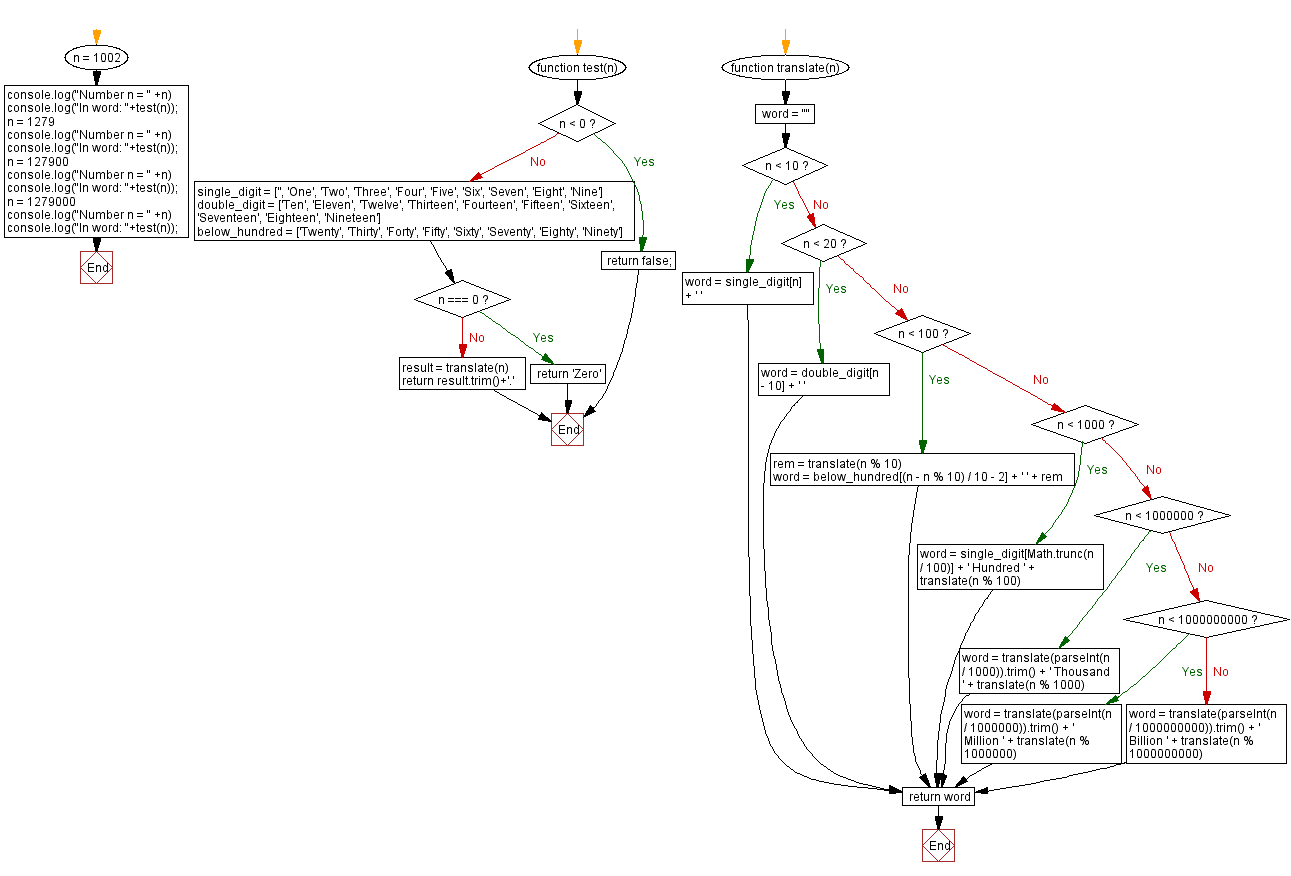# JavaScript: Conversion of integers to English words

## JavaScript Math: Exercise-105 with Solution

Write a JavaScript program that converts a given non-negative integer number to its English representation.

Test Data:
(1002) -> One Thousand Two.
(1279) -> One Thousand Two Hundred Seventy Nine.
(127900) -> One Hundred Twenty Seven Thousand Nine Hundred.
(1279000) -> One Million Two Hundred Seventy Nine Thousand.

Sample Solution:

HTML Code:

``````<!DOCTYPE html>
<html>
<meta charset="utf-8">
<title>JavaScript program to Conversion of integers to English words</title>
<body>

</body>
</html>
```
```

JavaScript Code:

``````function test(n) {
if (n < 0)
return false;
single_digit = ['', 'One', 'Two', 'Three', 'Four', 'Five', 'Six', 'Seven', 'Eight', 'Nine']
double_digit = ['Ten', 'Eleven', 'Twelve', 'Thirteen', 'Fourteen', 'Fifteen', 'Sixteen', 'Seventeen', 'Eighteen', 'Nineteen']
below_hundred = ['Twenty', 'Thirty', 'Forty', 'Fifty', 'Sixty', 'Seventy', 'Eighty', 'Ninety']
if (n === 0) return 'Zero'
function translate(n) {
word = ""
if (n < 10) {
word = single_digit[n] + ' '
}
else if (n < 20) {
word = double_digit[n - 10] + ' '
}
else if (n < 100) {
rem = translate(n % 10)
word = below_hundred[(n - n % 10) / 10 - 2] + ' ' + rem
}
else if (n < 1000) {
word = single_digit[Math.trunc(n / 100)] + ' Hundred ' + translate(n % 100)
}
else if (n < 1000000) {
word = translate(parseInt(n / 1000)).trim() + ' Thousand ' + translate(n % 1000)
}
else if (n < 1000000000) {
word = translate(parseInt(n / 1000000)).trim() + ' Million ' + translate(n % 1000000)
}
else {
word = translate(parseInt(n / 1000000000)).trim() + ' Billion ' + translate(n % 1000000000)
}
return word
}
result = translate(n)
return result.trim()+'.'
}

n = 1002
console.log("Number n = " +n)
console.log("In word: "+test(n));
n = 1279
console.log("Number n = " +n)
console.log("In word: "+test(n));
n = 127900
console.log("Number n = " +n)
console.log("In word: "+test(n));
n = 1279000
console.log("Number n = " +n)
console.log("In word: "+test(n));
```
```

Sample Output:

```Number n = 1002
In word: One Thousand Two.
Number n = 1279
In word: One Thousand Two Hundred Seventy Nine.
Number n = 127900
In word: One Hundred Twenty Seven Thousand Nine Hundred.
Number n = 1279000
In word: One Million Two Hundred Seventy Nine Thousand.
```

Flowchart:Live Demo:

See the Pen javascript-math-exercise-105 by w3resource (@w3resource) on CodePen.

Improve this sample solution and post your code through Disqus

What is the difficulty level of this exercise?

Test your Programming skills with w3resource's quiz.

﻿

## JavaScript: Tips of the Day

Shorten an array using its length property

A great way of shortening an array is by redefining its length property.

```let array = [0, 1, 2, 3, 4, 5, 6, 6, 8, 9]
array.length = 4
// Result: [0, 1, 2, 3]
```

Important to know though is that this is a destructive way of changing the array. This means you lose all the other values that used to be in the array.

Ref: https://bit.ly/2LBj213